Analysis 7A31   Main

#Grandiose Nostalgiaby Richard Pavlicek

Perhaps the most written-up deal in bridge history is the grand slam that decided the 1975 Bermuda Bowl, the “tournament of infamy” that rocked the bridge world. I won’t rekindle the nefarious side issues, which are readily available from many sources, including my May 2006 bidding poll Italy Wins in Bermuda. This look back will focus on bridge, or more specifically, the play of that fateful grand.

On the famous deal Italy bid to an egregious 7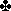, which was blessed with a lucky lie, allowing the contract to make. Had 7failed, North America would have won the Bermuda Bowl; and even on the fortuitous layout, a stronger American defense might still have defeated the grand. This was the deal.Board 92N-S Vul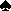Q J 8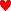A J 9 6 5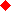K 8 2A Q KantarWestPassPassPassPassPassPassPass GarozzoNorth2344 NT557EisenbergEASTPassPassPassPassPassDblPassAll Pass BelladonnaSouth223 NT45Rdbl5 NT7 6 5 2K 4 3 2J 5 3K 10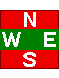4 3Q 10 8 7Q 10 6 47 5 4 7SouthLead:2A K 10 9—A 9 7J 9 8 6 3 2

The 2opening was natural (Precision) and 2was an artificial force. The next four bids were natural, then 4began a control-showing sequence; 4 NT was not Blackwood per Italian tradition, after which some of the actions are mysterious — not just to me but apparently to the Italians as well, considering the final bid.Eisenberg’s double of 5was strange, because his diamond holding suggests that lead would be more effective, perhaps setting up a trick before dummy’s heart suit could be established. Was he just antsy from passing too much? Conflicting accounts also have Eisenberg doubling 5, but this is incredulous; “Broadway” Billy may march to a different drummer, but he never lost his drumsticks.

Kantar dutifully led a heart, which Belladonna ruffed and led a club to the 10, queen. Game over!

Much speculation has been made on the outcome if Kantar had played the king on the first club lead, suggesting a singleton. Belladonna would then have an alternate line to exploit a trump coup against East’s presumed10-7-5-4. The catch of course is that it would be likely to fail if Kantar had played the king from a doubleton, a risk-free falsecard. Belladonna remarked to the media in the days after that he would have played for the coup (going down on the layout) but this may have been influenced by celebration and too much vino di Italia.

 Analysis 7A31   MainTop   Grandiose Nostalgia

##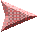A Closer Look

Recently a longtime cyber friend, Charles Blair, asked if I thought Belladonna should have played for the trump coup if theK had appeared. This is subjective of course, as it depends on how often theK is played from a doubleton. Assuming say 50-50, my gut reaction favored the coup line, since it would sometimes work against king-doubleton. Charles felt otherwise, convinced that drawing trumps was right. Considering Charles is a mathematician (retired, U. of Illinois) I didn’t like my chances, but it piqued my interest to do a detailed analysis.

For the first analysis I will ignore the double of 5(which shouldn’t happen anyway) and assume a nondescript diamond lead, the only unshown suit.* Declarer would win in hand with theA and lead a club (king, ace) then take one of two lines: (A) Cash theQ; if West shows out, East must be 4=3=2=4, else failure. (B) Cash theA (diamond pitch), ruff a heart, cross to theQ and ruff another heart. If either opponent shows out, win theQ (coup won’t work); else cash two spades ending in dummy, ruff a heart, cross to theK, ruff a diamond, then high-crossruffQ-J.

*The reason for changing the lead is to achieve an unbiased state. When a defender chooses to lead dummy’s suit it portends foul distribution, while choosing to lead the unshown suit is innocuous.

The following table compares Line A and Line B when theK is singleton, hence East holds10-7-5-4. For each case that allows success, the number of hands is calculated by combinatorics, and the totals show the number of hands on which each line succeeds.

CaseWestEastLine A SuccessLine B Success
11=5=6=15=3=1=400
21=6=5=15=2=2=400
32=4=6=14=4=1=400
42=5=5=14=3=2=415·56·21 = 1764015·56·21 = 17640
52=6=4=14=2=3=400
63=3=6=13=5=1=400
73=4=5=13=4=2=4020·70·21 = 29400
83=5=4=13=3=3=4020·56·35 = 39200
93=6=3=13=2=4=400
104=2=6=12=6=1=400
114=3=5=12=5=2=400
124=4=4=12=4=3=400
134=5=3=12=3=4=400
144=6=2=12=2=5=400
155=2=5=11=6=2=400
165=3=4=11=5=3=400
175=4=3=11=4=4=400
185=5=2=11=3=5=400
195=6=1=11=2=6=400
Total successful handsLine A = 17640Line B = 86240

To simplify the analysis I eliminated all East-West patterns with a singleton heart or any void. Besides being rare and of little significance, these cases would often evolve differently; e.g., a bid with 7+ cards in a red suit, or a Lightner double.

The next table compares Line A and Line B when theK is doubleton, which comprises four holdings as West can have K-10, K-7, K-5 or K-4. Line A always works. Line B only works with spades 3-3, or if a 6-2 heart break dictates abandonment.

CaseWestEastLine A SuccessLine B Success
201=4=6=25=4=1=36·70·7·4 = 117600
211=5=5=25=3=2=36·56·21·4 = 282240
221=6=4=25=2=3=36·28·35·4 = 235206·28·35·4 = 23520
232=3=6=24=5=1=315·56·7·4 = 235200
242=4=5=24=4=2=315·70·21·4 = 882000
252=5=4=24=3=3=315·56·35·4 = 1176000
262=6=3=24=2=4=315·28·35·4 = 5880015·28·35·4 = 58800
273=2=6=23=6=1=320·28·7·4 = 1568020·28·7·2 = 7840
283=3=5=23=5=2=320·56·21·4 = 9408020·56·21·3 = 70560
293=4=4=23=4=3=320·70·35·4 = 19600020·70·35·4 = 196000
303=5=3=23=3=4=320·56·35·4 = 15680020·56·35·4 = 156800
313=6=2=23=2=5=320·28·21·4 = 4704020·28·21·4 = 47040
324=2=5=22=6=2=315·28·21·4 = 3528015·28·21·2 = 17640
334=3=4=22=5=3=315·56·35·4 = 1176000
344=4=3=22=4=4=315·70·35·4 = 1470000
354=5=2=22=3=5=315·56·21·4 = 705600
364=6=1=22=2=6=315·28·7·4 = 1176015·28·7·4 = 11760
375=2=4=21=6=3=36·28·35·4 = 235200
385=3=3=21=5=4=36·56·35·4 = 470400
395=4=2=21=4=5=36·70·21·4 = 352800
405=5=1=21=3=6=36·56·7·4 = 94080
Total successful handsLine A = 1358672Line B = 589960

Line B notes: Cases 27 and 32 fail againstK-10 or K-7 (overruff) so the club factor is reduced to 2. Case 28 fails againstK-10 (overruff) so the club factor is reduced to 3.

The above tables show that Line A succeeds against 1358672 hands with king-doubleton, and 17640 hands with king-singleton. Line B succeeds against 589960 hands with king-doubleton, and 86240 hands with king-singleton. Let p = the probability of king-doubleton after the king is seen. The proportion of Line A success is then 1358672p + 17640(1-p), and Line B is 589960p + 86240(1-p). Equating the two will discover the value of p for which the lines are equivalent, hence:

1358672p + 17640(1-p) = 589960p + 86240(1-p)

Solution: p = 5/42, or approximately 0.119 or 11.9 percent

This means that if the king (once seen) would be doubleton 11.9 percent of the time, the lines are equal. Anything higher would favor Line A; anything lower would favor Line B.The above does not indicate how often the king should be played from a doubleton, but this can be calculated. The probability of a specific doubleton (missing five cards) is 39/1150, so the probability of any king-doubleton is 4×39/1150 = 78/575. Now suppose the king is played 1/t of the time, which makes the probability of king from king-doubleton (1/t)×(78/575) = 78/(575t). The probability of a singleton king is 13/460. Therefore, the ratio of king from king-doubleton to the whole (king from king-doubleton + singleton king) must equal the 5/42 probability obtained above, hence:

78/(575t)/(78/(575t)+13/460) = 5/42

Solution: t = 888/25, or 1/t = 25/888

This means that if the king is played 25/888 of the time from king-doubleton, the lines are equal. Anything more frequent (say 1/35 of time) would favor Line A; anything less frequent (say 1/36 of time) would favor Line B. Charles was certainly on the mark, as Line A is better if the falsecard is made as little as once in 35 times. The flaw in my initial assessment was to compare a blank king with the actual K-10 doubleton, rather than all king-doubletons, which quadruples the weight of those cases.

The conclusion is clear. In an expert game the falsecard would be made far more often than 1/35 of the time, probably over half the time, so playing for the coup is a long shot. Basic Bridge 101: Draw trumps!

 Analysis 7A31   MainTop   Grandiose Nostalgia

##After the Double

Now let’s consider the impact of Eisenberg’s double of 5. Does it affect the calculations? And if so, which line does it favor? And there’s also the real-life possibility that it may have affected Kantar, distracting him from his usual sterling play.Clearly the double affects the plausible heart distributions, of which East should have at least four. This is confirmed by West’s2 lead (fourth-best or low from three) which marks East with four or five hearts; hence all other heart layouts will be ignored. Further, East’s double must show strength in hearts, so I’ve halved the number of heart combinations to allow for the occasions where East would not be strong enough to double — hardly an exact adjustment but a good approximation.

The lines of play are altered slightly after a heart lead, but essentially the same. Declarer begins by ruffing the first trick and leading a club (king, ace) then Line A is the same. Line B only varies by cashing three rounds of spades after ruffing the third round of hearts, and cashing theA at some point along the way.

As before, the first table compares Line A and Line B when theK is singleton. Almost a wipeout, as the heart layout restrictions prevent Line A from ever working, and Line B only works in Case 7.

CaseWestEastLine A SuccessLine B Success
32=4=6=14=4=1=400
63=3=6=13=5=1=400
73=4=5=13=4=2=4020·35·21 = 14700
114=3=5=12=5=2=400
124=4=4=12=4=3=400
165=3=4=11=5=3=400
175=4=3=11=4=4=400
Total successful handsLine A = 0Line B = 14700

Case numbering is kept the same as in previous tables, but patterns with East having 2, 3 or 6 hearts (deemed impossible) are removed.

The next table compares Line A and Line B when theK is doubleton. Line A works against all cases deemed possible, while Line B succeeds only in Case 28 and 29.

CaseWestEastLine A SuccessLine B Success
201=4=6=25=4=1=36·35·7·4 = 58800
232=3=6=24=5=1=315·28·7·4 = 117600
242=4=5=24=4=2=315·35·21·4 = 441000
283=3=5=23=5=2=320·28·21·4 = 4704020·28·21·3 = 35280
293=4=4=23=4=3=320·35·35·4 = 9800020·35·35·4 = 98000
334=3=4=22=5=3=315·28·35·4 = 588000
344=4=3=22=4=4=315·35·35·4 = 735000
385=3=3=21=5=4=36·28·35·4 = 235200
395=4=2=21=4=5=36·35·21·4 = 176400
Total successful handsLine A = 380240Line B = 133280

Line B note: Case 28 fails againstK-10 (overruff) so the club factor is reduced to 3.

The revised tables show that Line A succeeds against 380240 hands with king-doubleton, and none with king-singleton. Line B succeeds against 133280 hands with king-doubleton, and 14700 hands with king-singleton. Plugging the new values into the first equation gives:

380240p = 133280p + 14700(1-p)

Solution: p = 5/89, or approximately 0.056 or 5.6 percent

This means that if the king (once seen) would be doubleton 5.6 percent of the time, the lines are equal. Anything higher would favor Line A; anything lower would favor Line B.

Applying the 5/89 probability to the second equation gives:

78/(575t)/(78/(575t)+13/460) = 5/89

Solution: t = 2016/25, or 1/t = 25/2016

Quite a difference. The lines are now equal if the king is played 25/2016 of the time from king-doubleton. Line A is better if the king is played at least once in 80 times; Line B is better if the king is played at most once in 81 times. Evidently Eisenberg’s double was even more wayward than it looked, diminishing the chance that Belladonna would have pursued the ill-fated coup if given the opportunity.

As a final note, appropriate for this Bermuda Bowl’s sideshows, on the first club lead Kantar should have tossed the king in the air exclaiming “Rabbi’s rule!” with Eisenberg adding “Don’t worry, pard, I’ve got you covered.”

 Analysis 7A31   MainTop   Grandiose Nostalgia